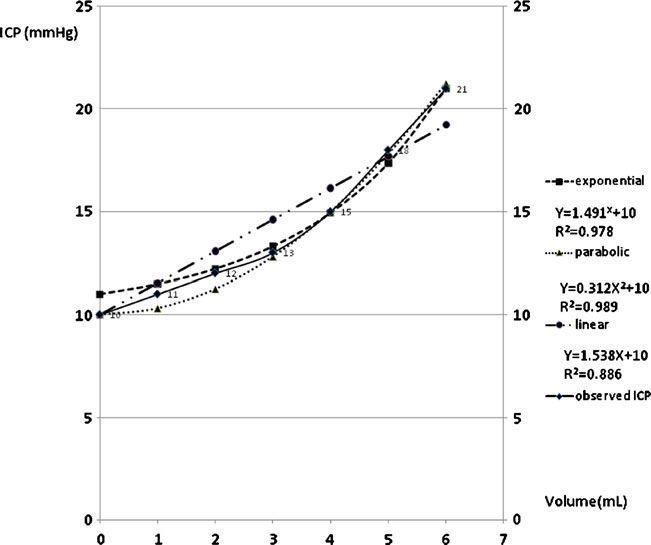# The intracranial volume pressure response in increased intracranial pressure patientsActa Neurochir (2012) 154:2271–2275

The intracranial pressure (ICP) is usually continuously monitored in the management of patients with increased ICP. The aim of this study was to discover a mathematic equation to express the intracranial pressure– volume (P–V) curve and a single indicator to reflect the status of the curve.

Methods Patients with severe brain damage who had bilateral external ventricular drainage (EVD) from December 2008 to February 2010 were included in this study. The EVD was used as drainage of CSF and ICP monitor. The successive volume pressure response values were obtained by successive drainage of CSF from ICP 20–25 to 10 mmHg. Parabolic, exponential, and linear regression models were designed to have a single parameter as the indicator to determine the P–V curves.

Results The mean of parameter “a” in the exponential equation is 1.473±0.054; in the parabolic equation, it is 0.332±0.061; and in the linear equation, it is 1.717±0.209. All regression equations of P–V curves had statistical significance (p<0.005). Parabolic and exponential equations are closer to the original ICP curve than linear equation (p< 0.005). There is no statistically significant difference between parabolic and exponential regressions.

Conclusions The P–V curve can be expressed with linear, parabolic, and exponential regression models in increased ICP patients. The parabolic and exponential equations are more accurate methods to represent the P–V curve. The single parameter in the three regression equations can be compared in different conditions of one patient in clinical practice.